# LIC AAO Pre Quantitative Aptitude Quiz-17

## LIC AAO Pre Quantitative Aptitude Quiz

Quantitative aptitude measures a candidate’s numerical proficiency and problem-solving abilities. It is the most important section of almost all competitive exams. Candidates are often stymied by the complexity of Quantitative Aptitude Questions but if they practice more and more questions it will become quite easy. So, here we are providing you with the LIC AAO Pre Quantitative Aptitude Quiz to enhance your preparation for your upcoming examination. Questions given in this LIC AAO Pre Quantitative Aptitude Quiz are based on the most recent and the latest exam pattern. A detailed explanation for each question will be given in this LIC AAO Pre Quantitative Aptitude Quiz. This LIC AAO Pre Quantitative Aptitude Quiz is entirely free of charge. This LIC AAO Pre Quantitative Aptitude Quiz will assist aspirants in achieving a good score in their upcoming examinations.

Direction (1-5): In each of these questions, two equations (I) and (II) are given. You have to solve both the equations and give answer.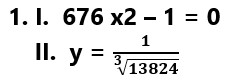(a)     If x > y

(b)     If x ≥ y

(c)     If x < y

(d)     If x ≤ y

(e)     If x = y or relationship between x and y cannot be established.1. I. 2×2 + 5x – 33 = 0

II. y2 – y – 6 = 0

(a)     If x > y

(b)     If x ≥ y

(c)     If x < y

(d)     If x ≤ y

(e)     If x = y or relationship between x and y cannot be established.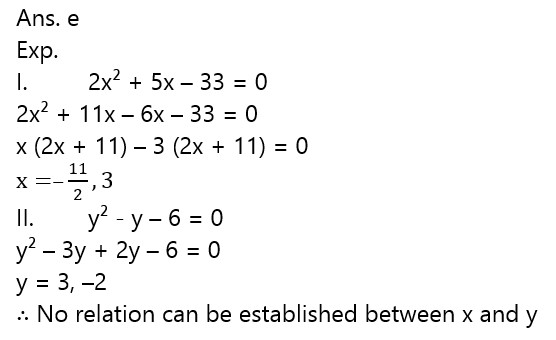1. I. x2 + 12x + 36 = 0

II. y2 + 15y + 56 = 0

(a)     If x > y

(b)     If x ≥ y

(c)     If x < y

(d)     If x ≤ y

(e)     If x = y or relationship between x and y cannot be established.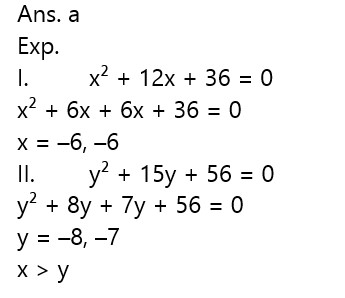1. I. 2×2 – 3x – 35 = 0

II. y2 – 11y + 30 = 0

(a)     If x > y

(b)     If x ≥ y

(c)     If x < y

(d)     If x ≤ y

(e)     If x = y or relationship between x and y cannot be established.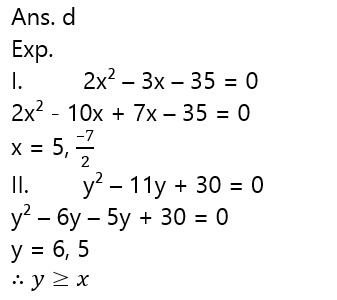1. I. 12×2 – 46x + 40 = 0

II. 4y2 + 3y – 10 = 0

(a)     If x > y

(b)     If x ≥ y

(c)     If x < y

(d)     If x ≤ y

(e)     If x = y or relationship between x and y cannot be established.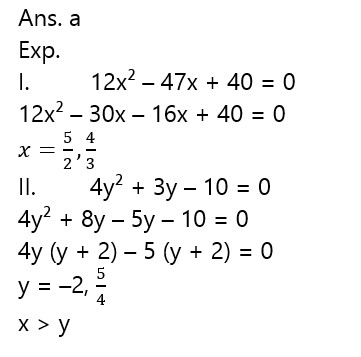Directions (6-10): Bar chart given below shows number of students studying in three different streams in four different colleges. Study the following graph carefully and answer the questions given below it.1. Find the difference between the total number of students studying in college H and that of studying in college K?

(a) 16100

(b) 15800

(c) 16300

(d) 16700

(e) None of these

Ans. d

Exp.

Total number of student studying in college H = 51,200 + 40,000 + 36,500 = 1,27,700

Total number of student studying in college K = 30,000 + 56,000 + 25,000 = 1,11,000

Required difference = 1,27,700 – 1,11,000 = 16,700

1. Find total number of students in commerce stream studying in all the colleges together?

(a) 1,58,500

(b) 1,20,500

(c) 1,60,500

(d) 1,10,500

(e) 1,54,500

Ans. e

Exp.

Student in Commerce stream studying in all college together

= 36,500 + 33,000 + 60,000 + 25,000

= 1,54,500

1. Find the ratio between the students in Science stream in college H and I together to the students in the same stream in college J and K together?

(a) 43 : 45

(b) 41 : 43

(c) 45 : 43

(d) 43 : 41

(e) 45 : 41

Ans. c

Exp.
Students in Science stream in colleges H and I together
= 40000 + 50000 = 90000

Students in Science stream from college J and K together
= 30000 + 56000 = 86000
Required ratio =90,000/86,000=  45/43

1. Total number of students in Science stream in college I is approximately what per cent of the total number of students studying in same stream all the colleges together?

(a) 28%

(b) 38%

(c) 24%

(d) 34%

(e) 44%

Ans. a
Exp.
Students in science stream in college I = 50,000
Total students in science stream in all the colleges together = 40000 + 50000 + 30000 + 56000 = 176000
Required % =  50000/176000×100 ≈ 28%
1. Find the difference between average number of students in Art stream to average number of students in Science stream in all the colleges together?

(a) 17750

(b) 14200

(c) 10650

(d) 7100

(e) 3550